Enlightened

# Question 20: NCERT Solutions for 12th Class Physics: Chapter 7-Alternating Current

• 0

Question 20: NCERT Solutions for 12th Class Physics: Chapter 7-Alternating Current

A series LCR circuit with L = 0.12 H, C = 480 μF, R = 23 Ω2 is connected to a 230 V variable frequency supply.
(a) What is the source frequency for which current amplitude is maximum. Obtain this maximum value.
(b) What is the source frequency for which average power absorbed by the circuit is maximum. Obtain the value of this maximum power.
(c) For which frequencies of the source is the power transferred to the circuit half the power at resonant frequency? What is the current amplitude at these frequencies?
(d) What is the Q-factor of the given circuit?

Share

1. Solution: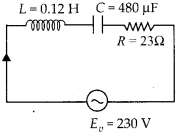(a) At resonant frequency, the current amplitude is maximum.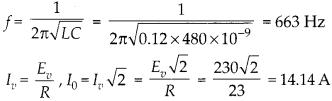(b) Maximum power loss at resonant frequency, P = Eυlυ cos θ(c) Let at an angular frequency, the source power is half the power at resonant frequency.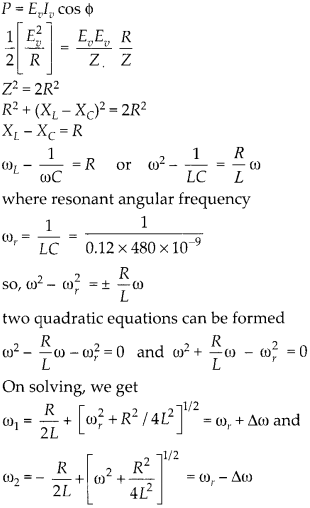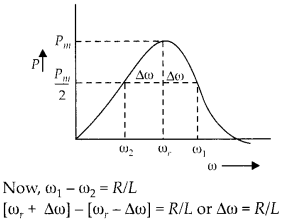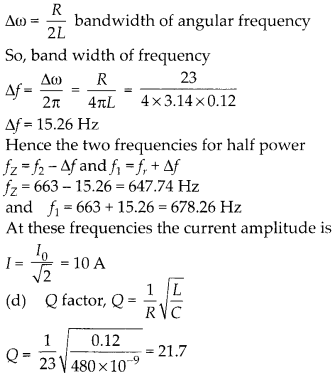Check the complete chapter with solutions.

NCERT Solutions for 12th Class Physics: Chapter 7-Alternating Current

• 0